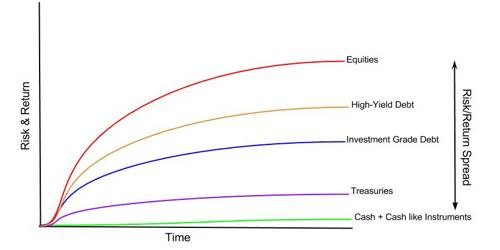# Risk Premium

Risk Premium: According to Beasley and Brigham, “The portion of the expected return that can be attributed to the additional risk of an investment it is the difference between the expected rate of return on given risky assets and on a less risky asset. For example, high-quality corporate bonds issued by established corporations earning large profits typically have very little risk of default.

According to Ross, Westerfield, and Jordan, “The excess return required from an investment in risky assets over that required from a risk-free investment.” The concept of a risk premium is used mostly by investors and finance students studying and dealing with the financial markets. It may also be a measure of the extra return that an investor demands to bear risk – a market portfolio’s reward-to-risk ratio.

RP = E(R) – Rf.

Where, Rp = Risk premium.

E(R) Expected return on the market portfolio.

Rf = Risk-free rate of return.

Example; Assume the risk-free rate is 5%. This means a riskless government treasury bond offers an annual return of 5%. Let’s say an investor invests in the stock of a company and that stock has an annual return of 7%. The risk premium for that company’s stock is the difference between the risk-free rate of 5% and the expected return of the stock of 7%. So the risk premium is 2%.

Risk Premium = Asset Return – Risk-Free Rate = 2% = 7% – 5%

We can see that Risk-free premium is got by deducting risk-free income from an average of portfolio return. The risk premium of the market is the average return on the market minus the risk-free rate.